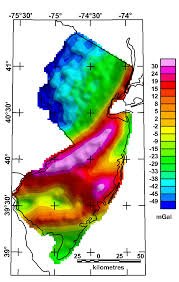## How to Calculate and Solve for Bouguer Anomaly | GravityThe image above represents bourger anomaly.

To compute for bourger anomaly, three essential parameters are needed and these parameters are Free Air Correction (gF), Bouguer Correction (δgB) and Terrain Correction (δgT).

The formula for calculating bourger anomaly:

gB = gF – δgB + δgT

Where:

gB = Bouguer Anomaly
gF = Free Air Correction
δgB = Bouguer Correction
δgT = Terrain Correction

Let’s solve an example;
Find the bourger anomaly when the free air correction is 14, the bourger anomaly is 18 and the terrian correction is 20.

This implies that;

gF = Free Air Correction = 14
δgB = Bouguer Correction = 18
δgT = Terrain Correction = 20

gB = gF – δgB + δgT
gB = 14 – 18 + 20
gB = 14 – 38
gB = -24

Therefore, the bourger anomaly is -24 mGal.